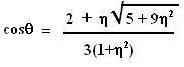## Thursday, December 6, 2007

### Irodov Problem 1.108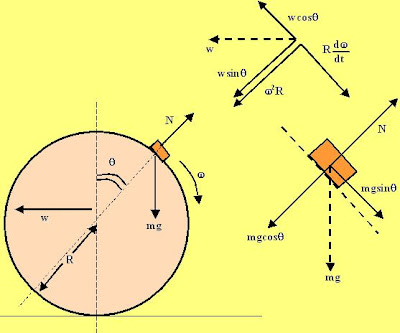Suppose that the mass of the body is m and let the instantaneous angular velocity of the body iswith respect to a frame on the center of the sphere (ie. as seen by someone at the center of the sphere). At any instant of time, there are two forces acting on this body - i) the normal reaction from the surface of the sphere and ii) the force of gravity. The net acceleration of the body with respect to a stationary observer can be expressed as the sum of two parts, i) acceleration as seen from the observer at the center of the sphere and ii) the acceleration of the sphere itself (w).

The force diagram is shown in the figure. Let us consider the forces and accelerations in the directions normal and tangential to the surface when the radius vector of body from the center of the sphere is at an angle.

Direction Normal to the Surface
There are two forces in this direction i) the Normal reaction N and ii) the component of gravity. The acceleration of the body as seen by an observer at the center of the sphere in this direction is simply the centripetal acceleration directed radially inwards given by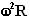. The component of the spheres acceleration in this direction is given by. The net acceleration of the body in this direction with respect to a stationary observer is thus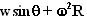. Hence we can write,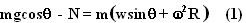Direction tangential to the surface
There is only one force acting in this direction namely the component of force of gravity.
The tangential acceleration of the body as seen by the observer at the center of the sphere is. The component of acceleration of the sphere itself along this direction is given by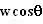. Thus, the net acceleration of the body with respect to a stationary observer is given by. Hence we have ,Now we can use equations (1) and (2) to solve rest of the problem.Now we can use (3) in (1) to get,When the body just leaves the surface, N=0 and so from (5) we have,The velocity of the body relative to the sphere is essentially the tangential velocity given by,(b) For this part we simply set N=0 in (4) and have,We neglect the - sign and take the higher value since this means the smaller of the two angles (cosine function decreases with increasing angles). Thus,